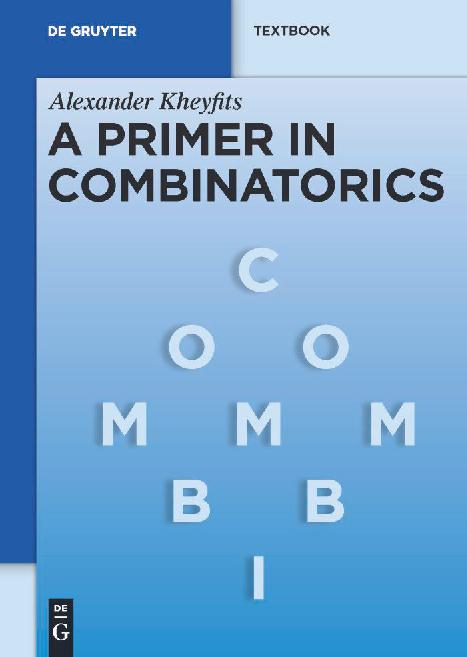### Book Toankho

Sách hay - Sách đẹp

# A Primer in Combinatorics (de Gruyter Textbook)This textbook is devoted to Combinatorics and Graph Theory, which are cornerstones of Discrete Mathematics. Every section begins with simple model problems. Following their detailed analysis, the reader is led through the derivation of definitions, concepts and methods for solving typical problems. Theorems then are formulated, proved and illustrated by more problems of increasing difficulty. Topics covered include elementary combinatorial constructions, application to probability theory, introduction to graphs and trees with application to hierarchical clustering algorithms, more advanced counting techniques, and existence theorems in combinatorial analysis. The text systematically employs the basic language of set theory. This approach is often useful for solving combinatorial problems, especially problems where one has to identify some objects, and significantly reduces the number of the students´ errors; it is demonstrated in the text on many examples. The textbook is suitable for undergraduate and entry-level graduate students of mathematics and computer science, lecturers in these fields, and anyone studying combinatorial methods and graphical models for solving various problems. The book contains more than 700 problems and can be used as a reading and problem book for an independent study seminar or self-education.